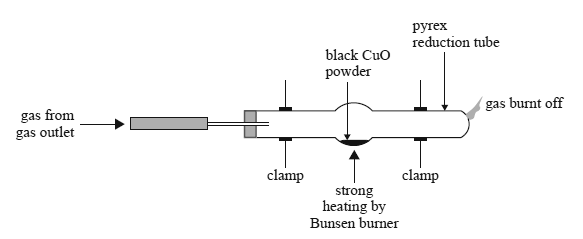General (2014 VCE) 1) When hydrochloric acid is added to aluminium sulfide, the highly toxic gas hydrogen sulfide is evolved. The equation for this reaction is Al2S3(s) + 6HCl(aq) => 2AlCl3(aq) + 3H2S(g) If excess hydrochloric acid is added to 0.200 mol of aluminium sulfi de, then the volume of hydrogen sulfide produced at standard laboratory conditions (SLC) will be A. 1.63 L B. 4.90 L C. 7.35 L D. 14.7 L Solution2) An aerosol can with a volume of 300.0 mL contains 2.80 g of propane gas as a propellant. The warning label says the aerosol may explode at temperatures above 60.0 °C. What is the pressure in the can at a temperature of 60.0 °C? A. 5.87 × 10–1 kPa B. 1.06 × 102 kPa C. 5.87 × 102 kPa D. 2.58 × 104 kPa Solution3) If Solution X has a pH of 3 and Solution Y has a pH of 6, we can conclude that A. [H+] in Solution X is 1000 times that of [H+] in Solution Y. B. [H+] in Solution X is half that of [H+] in Solution Y. C. [OH-] in Solution Y is twice that of [OH-] in Solution X. D. Solution Y must contain a stronger acid than Solution X. Solution4) What volume of 0.25 M hydrochloric acid is required to react completely with 40 mL of 0.50 M calcium hydroxide? A. 40 mL B. 80 mL C. 120 mL D. 160 mL Solution5) Which one of the reactions of hydrochloric acid below is a redox reaction? A. 2HCl(aq) + Fe(s) =>H2(g) + FeCl2(aq) B. 2HCl(aq) + Na2S(s) => H2S(g) + 2NaCl(aq) C. 2HCl(aq) + MgO(s) => MgCl2(aq) + H2O(l) D. 2HCl(aq) + K2CO3(s) => CO2g) + 2KCl(aq) + H2O(l)   Solution6) Consider the following unbalanced ionic equation. Hg(l) + Cr2O72–(aq) + H+(aq) => Hg2+(aq) + Cr3+(aq) + H2O(l) When this equation is completely balanced, the coefficient of Hg(l) will be A. 1 B. 2 C. 3 D. 4 Solution7) Which one of the following statements about 10.0 mL of 0.10 M HCl and 10.0 mL of 0.10 M CH3COOH solutions is true? A. Each solution will have the same electrical conductivity. B. Each solution will react completely with 10.0 mL of 0.10 M NaOH solution. C. Each solution will react at the same rate with 1.00 g of magnesium ribbon. D. The concentration of H3O+ ions will be greater in the CH3COOH solution Solution8) Some students conducted an experiment to determine the percentage by mass of copper in copper(II) oxide. The apparatus they used is shown in the diagram below.The equation for the redox reaction is 2CuO(s) => 2Cu(s) + O2(g) The gas passing through the tube prevented the copper from re-oxidising to CuO. The students weighed: • the empty tube • the tube and CuO before heating • the tube and Cu after heating and cooling. They found that the percentage by mass of copper in the copper oxide was 76.42%. The theoretical value is 79.86%. Which one of the following could not be a possible explanation for the lower experimental result? A. The copper(II) oxide, which is black, was contaminated with some carbon. B. Some copper(II) oxide remained unreacted when heating was stopped. C. Contamination on the outside of the tube was burnt off during the heating. D. Some of the copper(II) oxide powder was blown out of the tube by the gas. Solution 9) Consider the following information about the reaction of Ru2+ with various reagents. Ru2+(aq) + Fe2+(aq) => no observed reaction Ru2+(aq) + Ni(s) => Ru(s) + Ni2+(aq) Ru2+(aq) + Ag(s) => no observed reaction Ru2+(aq) + Cu(s) => Ru(s) + Cu2+(aq) Where would the following reaction be placed in the electrochemical series if the above tests were carried out under standard conditions? Ru2+(aq) + 2e– => Ru(s) A. below –0.23 V B. between –0.44 V and –0.23 V C. between 0.77 V and 0.34 V D. above 0.77 V Solution